Project tutorial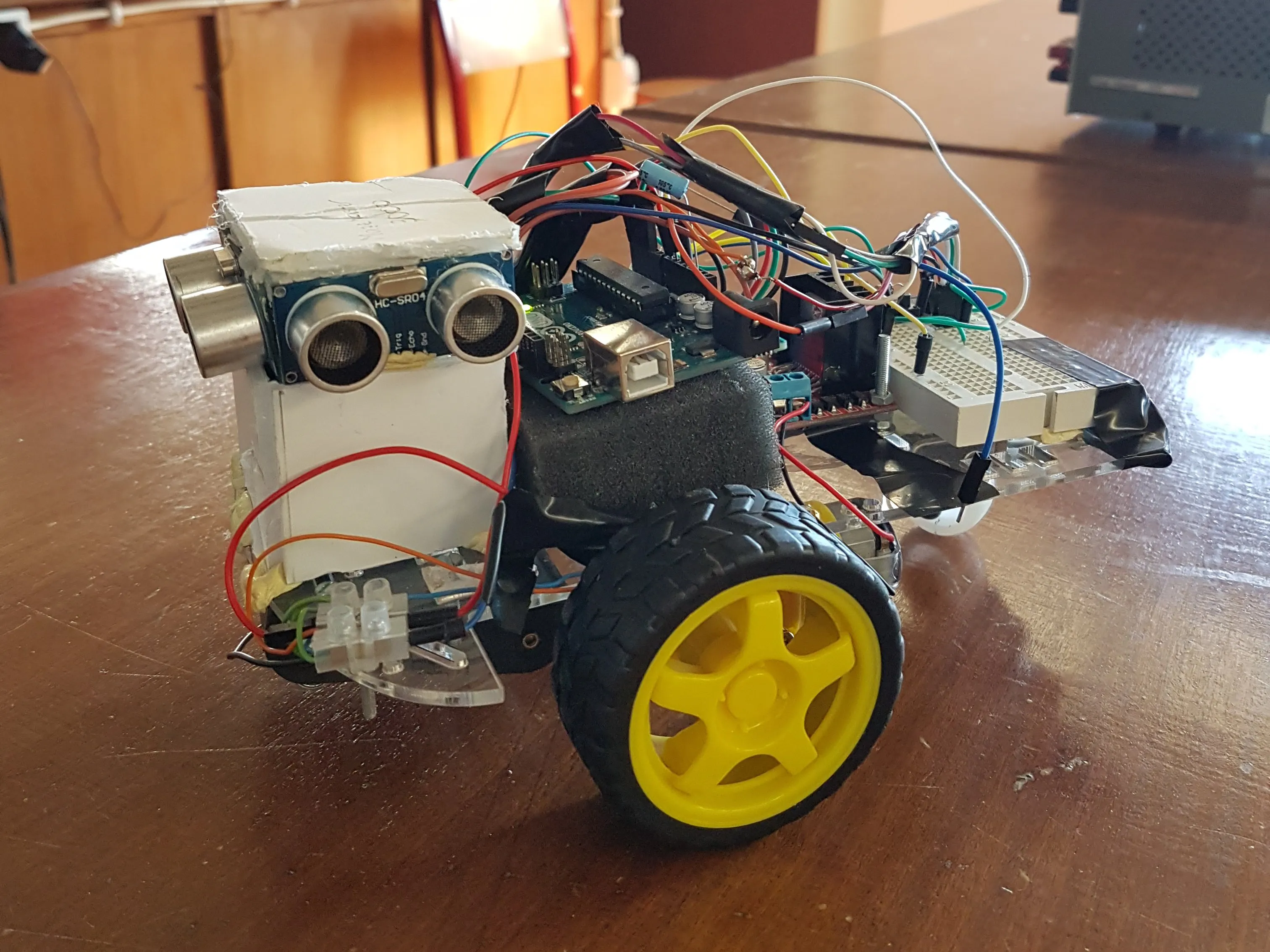# Mazemaster3000 © GPL3+

A finite state machine-powered maze solver that stores a log of steps without any external storage device.

• 997 views
• 6 respects

## Components and suppliesArduino UNO & Genuino UNO
×1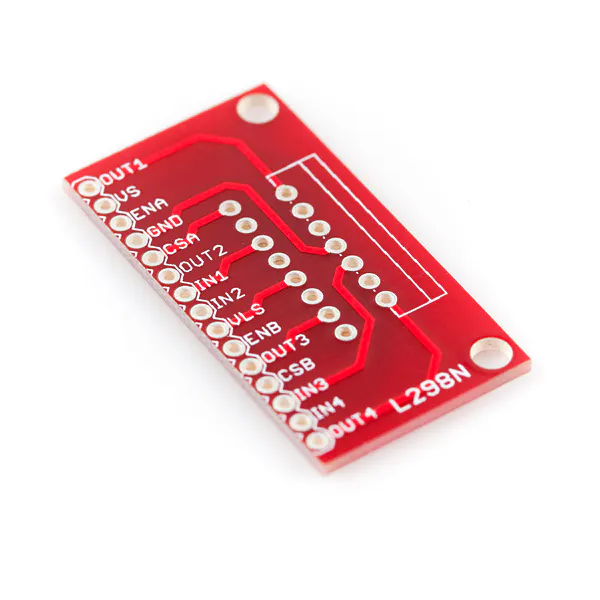SparkFun Full-Bridge Motor Driver Breakout - L298N
×1Resistor 10k ohm
×1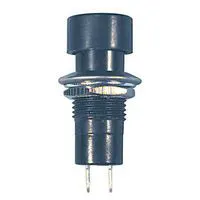Pushbutton Switch, Momentary
×1Ultrasonic Sensor - HC-SR04 (Generic)
×3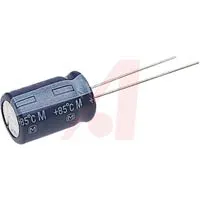Capacitor 10 µF
×1
 Robotic car kit
×1Jumper wires (generic) Just make sure to have a bunch of them, of all kinds
×1AA Batteries
×1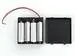4xAA battery holder
×1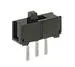Slide Switch
×1

## Necessary tools and machinesHot glue gun (generic)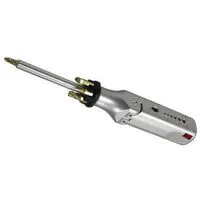Multitool, Screwdriver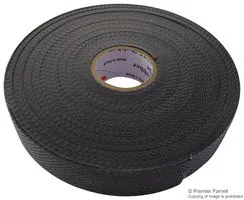Tape, Electrical

This project is being posted on behalf of Gonçalo Azinheira and João Castro, students at the University of the Algarve (Ualg).

It was conceived and made as a final project for the Microprocessors class.

It uses a finite state machine for of the decision making, which leads from either left corner of a square maze to a 2x2 open square on either corner of the right, which it will perceive as the ending.

It works on cell-based labyrinth. This means that mazes he runs are thought to have a sort of grid, meaning it goes from 1 square cell to the next, the barriers being in between cells.

At the end of the course, it flashes the Arduino's builtin LED and waits to be connected to a computer, so that it can print all of the steps it made on the serial monitor.

The following pictures comprise the entity, architecture, sub-architecture and the finite state machine.

In the entity we have the two In's that the h bridge uses to control each pole of the two motors and the En that controls the velocity through a pwm, an array which stores the log and the LED of the arduino being controlled using the pushbutton and the values measured from the pingers.

The architecture shows how the FSM function manages everything excluding the input from the pushbutton which is received as an external interruption.

In the sub-architecture we can see how the FSM function calls the various functions that analyse the information coming in:

-ping() sends various trigger signals through the pinger, receives the echoes and calculates, using the elapsed time, the distance from itself to the nearest obstacle in that direction, returning a mean of the various measurement values;

• pings() returns an int from 1 to 4 going right, front, left, back, according to what is direction is available. It starts measuring the right, measures front if it is under the set value, and left if that's not available. This kind of thought process always solves the maze;
• walk() makes the car go front a set time, back() turns the car 180º, turnR() turns 90º to the right and takes a step and turnL() turns 90º to the left and takes a step;
• Recb() prints rec[], which is the log of the steps taken. 1 means it turn to it's relative right and went to the cell it was facing(the cell that was directly to the right), 2 means it went directly to the cell it was facing, 3 is analogous to 1 but to the left and 4 means he turn around. 5 means he finished. This happens when he makes three lefts in a row(rec[] will always have three 3's before 5);

The click of the button starts FSM and, when it finishes, you are intended to connect the 10uF capacitor which is connected to reset to the GND, connect the car to your computer via USB, open the serial monitor and press the button again. This will print its log.

## Code

##### MazeMaster.inoC/C++
```//Authors: Gonçalo Azinheira and João Castro, students at University of the Algarve(Ualg);
//Project: Mazemaster3000;
//Subject: A finite state machine-powered maze solver. The starting point of the maze must be in one of the left corners and the ending must be in one of the right corners.

//----------------------------------------------------------------------------

const int pingt = 7; //the same trigger is used by all sensors
const int pinge2 = 6; //front ultrassonic sensor
const int pinge1 = 8; //right ultrassonic sensor
const int pinge3 = 9; //left ultrassonic sensor
int rec;
int a;
int In1 = A5; //right
int In2 = A4; //right
int In3 = A3; //left
int In4 = A2; //left
int EnA = 10; //right
int EnB = 11; //left
int dir;
int esqdist;
int dirdist;
int fdist;
boolean button=0;

void B_ISR(){ //The button is activated by an Interrupt System Routine
button=1;
}

void setup() {
Serial.begin(9600);
//button=0;
pinMode(button, INPUT);
attachInterrupt(0,B_ISR,RISING);

pinMode(pingt, OUTPUT);
pinMode(pinge1, INPUT);
pinMode(pinge2, INPUT);
pinMode(pinge3, INPUT);
a=0;
pinMode(In1, OUTPUT);
pinMode(In2, OUTPUT);
pinMode(In3, OUTPUT);
pinMode(In4, OUTPUT);
pinMode(EnA, OUTPUT);
pinMode(EnB, OUTPUT);
pinMode(LED_BUILTIN, OUTPUT);
analogWrite(EnA, 200);
analogWrite(EnB, 200);
}

//******************************************
//---------------------------------------------------
int ping (int pinge){
long duration, cm;
int x,xmed;
int dela=25;
int MAX = 1500;
int num = 0;
int sum=0;

for(int i=0;i<=5;i++){
digitalWrite(pingt, LOW);
delayMicroseconds(2);
digitalWrite(pingt, HIGH);
delayMicroseconds(5);
digitalWrite(pingt, LOW);
duration = pulseIn(pinge, HIGH);
x = microsecondsToCentimeters(duration);
if(x<MAX){
num ++;
}
else{x=0;}
sum+=x;

delay(dela);
}

xmed=sum/num;
return xmed;
}

long microsecondsToCentimeters(long microseconds) {
// The speed of sound is 340 m/s or 29 microseconds per centimeter.
// The ping travels out and back, so to find the distance of the object we
// take half of the distance travelled.
return microseconds / 29 / 2;
}

//-----------------------------------------------
//**********************************
//Each funcion uses different values in the analogWrite and delay. That's because the motors aren't exactly the same and the battery will slightly lose power.

void walk(int wtime) //Makes the cars go straight
{
analogWrite(EnA, 173);
analogWrite(EnB, 171);
digitalWrite(In1, HIGH);
digitalWrite(In2, LOW);
digitalWrite(In3, HIGH);
digitalWrite(In4, LOW);
delay(wtime*1.8);
digitalWrite(In1, LOW);
digitalWrite(In2, LOW);
digitalWrite(In3, LOW);
digitalWrite(In4, LOW);
delay(5);
}

void turnR(int wtime) //Makes the car turn right and then go straight
{
analogWrite(EnA, 192);
analogWrite(EnB, 172);
digitalWrite(In1, LOW);
digitalWrite(In2, HIGH);
digitalWrite(In3, HIGH);
digitalWrite(In4, LOW);
delay(wtime-141);
analogWrite(EnA, 170);
analogWrite(EnB, 170);
digitalWrite(In1, HIGH);
digitalWrite(In2, LOW);
digitalWrite(In3, HIGH);
digitalWrite(In4, LOW);
delay(wtime*2+50);
digitalWrite(In1, LOW);
digitalWrite(In2, LOW);
digitalWrite(In3, LOW);
digitalWrite(In4, LOW);
delay(5);
}

void turnL(int wtime) //Makes the car turn left and then go straight
{
analogWrite(EnA, 170);
analogWrite(EnB, 170);
digitalWrite(In1, HIGH);
digitalWrite(In2, LOW);
digitalWrite(In3, LOW);
digitalWrite(In4, HIGH);
delay(wtime-130);
analogWrite(EnA, 170);
analogWrite(EnB, 170);
digitalWrite(In1, HIGH);
digitalWrite(In2, LOW);
digitalWrite(In3, HIGH);
digitalWrite(In4, LOW);
delay(wtime*2+30);
digitalWrite(In1, LOW);
digitalWrite(In2, LOW);
digitalWrite(In3, LOW);
digitalWrite(In4, LOW);
delay(5);
}

void back(int wtime) //Makes the car turn around 180. This happens when the sensor detect obstacles in all directions.
{
analogWrite(EnA, 170);
analogWrite(EnB, 170);
digitalWrite(In1, LOW);
digitalWrite(In2, HIGH);
digitalWrite(In3, HIGH);
digitalWrite(In4, LOW);
delay(wtime+220);
digitalWrite(In1, LOW);
digitalWrite(In2, LOW);
digitalWrite(In3, LOW);
digitalWrite(In4, LOW);
delay(5);
}

//**********************************
//-----------------------------------------------

//This is the implementation of the finit state machine shown in the picture. It starts always in state -1 and stays there until the button is pressed. After that, it will go to state 0
//and measures the distances using the ultrassonic sensors using the pings() function. According to what it measured, the finite state machine will go to the corresponding state using the
//dir value (the meaning of this variable is explained below in the pings() function). The states 1 to 4 are responsible for making the car turn right, go straight, turn left or turn around respectively.
//At each of those states, the first thing that is done is record a value in the array rec []. This value is equal to the the present state of the machine. For example, if the car is going
//straight ahead, it is in state 2 and the value recored in the array will be also 2. In other words, the array stores the actions done by the car to be written in the function Recb().
//The cars reaches to the end if it does 3 lefts in a row and to do so, it needs to go to state 3, state 8 and 5 consecutively. So state 8 represents the 2nd left and state 5 represents the
//3rd left. After reaching to the end of the maze, it goes from state 5 to 6. By this point, the finite state machine will be always moving from state 6 to 7 and vice-versa.
//State 6 turns on the arduino's builtin LED and state 7 turns it off. Regardless of if it is in 6 or 7, it waits for the button to be pressed to print the log.

void MazeMaster_FSM(){
static int state=-1; //The initial state is always -1 until the button is pressed.
const int D = 20;  //This is the reference distance, in cm, for the car to consider the obstacles. If the sensor detects something below this distance, it will consider as an obstacle.
const int wtime = 500;  //Time used on each action(right, straight, left or turn around).

switch(state){

case -1:
if(button==1)
{button=0;
state=0;}
break;

case 0:
dir=pings();
state=dir;
break;

case 1:
Serial.print("state = ");
Serial.println(state);
rec[a]=1;
a++;
turnR(wtime);

dir=pings();
state=dir;
break;

case 2:
Serial.print("state = ");
Serial.println(state);
rec[a]=2;
a++;
Serial.print("a2 = ");
Serial.println(a);
walk(wtime);

dir=pings();
state=dir;
break;

case 3:
Serial.print("state = ");
Serial.println(state);
rec[a]=3;
a++;
turnL(wtime);

dir=pings();
if(dir==3)state=8;
else state=dir;
break;

case 4:
Serial.print("state = ");
Serial.println(state);
rec[a]=4;
a++;
Serial.print("a4 = ");
Serial.println(a);
back(wtime);

dir=pings();
state=dir;
break;

case 5:
Serial.print("state = ");
Serial.println(state);
rec[a]=3;
a++;
turnL(wtime);
Serial.println("I HAVE ARRIVED! ");
rec[a]=5;
state=6;
break;

case 6:
digitalWrite(LED_BUILTIN,HIGH);
if(button==1)
{Recb();
button==0;}
delay(500);
state=7;
break;

case 7:
digitalWrite(LED_BUILTIN,LOW);

if(button==1)
{Recb();
button==0;}
delay(500);
state=6;
break;

case 8:
Serial.print("state = ");
Serial.println(state);
rec[a]=3;
a++;
turnL(wtime);

dir=pings();
if(dir==3)state=5;
else state=dir;
break;

}

}
//------------------------------

void Recb(){
Serial.print("start -> ");
for(int i=0;i<=a;i++)
{Serial.println(rec[i]);}
button=0;
}
//---------------------------

//This is the function that measures the distances using the ultrassonic sensors.
//As the ending of the maze is always in the right corner, the function will give priority the right . It will only start measuring the front distance
//if the right sensor detects an obstacle (values below 20cm). The same goes for the left sensor, it will only start measuring if the right and front sensors detect an obstacle.
//This type of functioning prevents the car to waste time in measuring distances that aren't be necessary.
//The function pings() returns a value, called dir, between 1 and 4. 1 - No obstacle on the right, 2 - Obstacle on the right, 3 - Obstacles in front and right and 4 - Obstacles in all directions.

int pings(){
const int D = 20;

dirdist = ping(pinge1);
Serial.print("distance at right(->) = ");
Serial.println(dirdist);
if(dirdist>D){dir=1;}
else{
fdist = ping(pinge2);
Serial.print("distance at front(^) = ");
Serial.println(fdist);
if(fdist>D){dir=2;}
else{
esqdist = ping(pinge3);
Serial.print("distance at left(<-) = ");
Serial.println(esqdist);
if(esqdist>D){dir=3;}
else {dir=4;}
}
}
return dir;
}

//---------------------------

void loop() {
MazeMaster_FSM();
}

```

## Schematics

To finish you should take the L298N, remove the jumpers in EnA and EnB and connect 5V to Arduino Vin, GND to Arduino GND, OUT1 & 2 to each of the poles of motor A(right) and OUT3&4 to each of the poles of motor B(left)
EnA and EnB should be on Arduino digital pins 10 and 11 respectively. They MUST be on pwm pins and 10 and 11 have the same frequency, so this shouldn't be tinkered with.
In1, 2, 3 and 4 should be on Arduino ANALOG PINS 5, 4, 3 and 2 respectively.

WARNING:The capacitor connection to ground MUST be disconnected when uploading or it will NOT upload. Connect BEFORE connecting to the computer after the Arduino's BUILTIN LED starts blinking (after 3 lefts).July 5, 2019

#### Members who respect this project

See similar projects
you might like

#### No Candy For YOU!

Project tutorial by Jonathan Tindal

• 1,194 views
• 7 respects

#### Automatic Fear 1.0

Project showcase by Alexis Santiago Allende

• 1,345 views
• 9 respects

#### Simple Programmable Robotic Arm

Project showcase by Ryan Chan

• 74,129 views
• 193 respects

#### Otto DIY+ Arduino Bluetooth Robot Easy to 3D Print

Project tutorial by Team Otto builders

• 63,989 views
• 198 respects

#### GPS Datalogger, Spatial Analysis, and Azure IoT Hub.

Project tutorial by Shawn Cruise

• 20,940 views